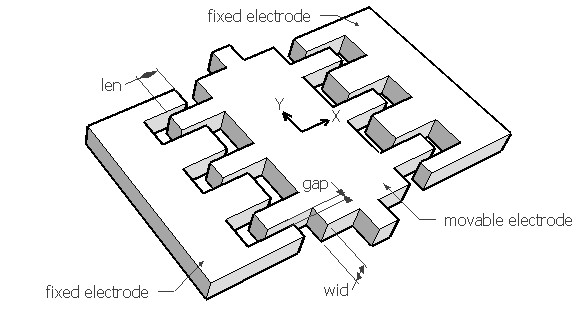COMBDR,len,wid,nf,gap,volt,k,rper,sel
Electrostatic comb drive actuator for lateral motion

len         overlapping length of the finger in µm
wid         width of finger in µm
nf           number of active fingers
gap        distance between the movable electrode and stationary electrode in µm
volt        applied voltage in Volt
k            lateral spring constant for X axis movement in N/m
rper       relative permittivity of the medium, it is approximately 1 for air
sel         number denoting the selected result.
Use 1 for balanced displacement in X, 2 for actuation force in X and 3 for minimum spring constant in Y directionNotes

The comb drive actuator typically consists of a movable electrode consisting of one or more finger like features forming a interdigitated comb like pattern with a fixed electrode. The interdigitated pattern ensures that the normal force between the electrodes in Y direction are canceled out. When a voltage is applied between the movable electrode and one fixed electrode, based on which fixed electrode is activated, the movable electrode will move laterally in X direction towards it. This movement will continue till the tangential electrostatic force due to all the active fingers are balanced by the elastic force of the spring suspension. This position is the balanced displacement and the corresponding electrostatic force is the actuation force. Actuation force is independent of the overlapping distance. The spring suspension is not shown in the figure, but the comb drive is designed to move in X direction and should be prevented from moving in Y direction. So the spring constant should be calculated for Y axis and based on that the beam design should be done. The movable electrode in the picture has six fingers, only three of which on one side will be activated at one time.

Using this design form, the balanced displacement, the actuation force and the minimum spring constant to prevent Y axis movement can be estimated. Assuming the supply voltage is the critical voltage that would force the fingers to move in Y direction, the minimum spring constant required in Y axis is calculated. If the spring constant is below this value, for the given voltage, the movable electrode will become unstable and move in Y axis till it hits the fixed electrode. Keeping the spring constant in Y above this value would ensure that the comb-drive is stable.

The plot shows the relationship between the applied voltage and the lateral displacement for the given comb drive. Using the crosshair tool, the displacement for any given voltage upto 20V can be estimated.

Assumptions

-The stationary electrodes are arranged symmetrically on both sides of the movable finger.
-The supply voltage is constant and there is no overshooting due to electrostatic forces.
-The medium between the electrodes is air by default with a relative permittivity of 1.
-The gap between the stationary electrodes and movable fingers is uniform.
-The spring constant of the beam is known. If unknown, this can be calculated under Mechanics > Structures > Beams.
-Damping is not considered.
-Parasitic capacitance is not considered.
-Constant driving voltage is assumed.